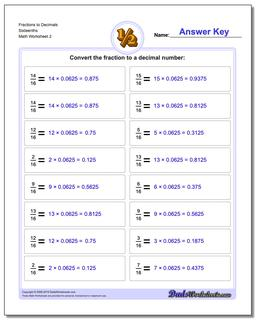# Math Worksheets: Fractions as Decimals: Fractions as Decimals: Fractions to Decimals Sixteenths (Second Worksheet)## Fractions to Decimals Sixteenths (Second Worksheet)

PropertyValue
DescriptionFractions to Decimals Sixteenths: Drills for converting common fractions into decimal equivalents. (Second Worksheet)
Resource TypeWorksheet